#### How to display a single quote in a cell?

In this Excel lesson you will teach yourself how to deal with single quote issues.

In Excel there is a problem that ‘yourtext’ (text inside single quotes) displays without leading single quote. There is an easy way to deal with this issue.

2. Choose Format Cells to format a data type for the cell.
3. Click Custom Category.
4. Write down ‘@ (single quote and at) as a type.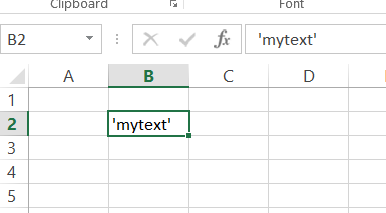That’s it. Your single quotes are displaying correctly since now.

## How to quote a quote in Excel formula?

Excel formulas are used to do some simple calculations, to drive results using other cell values and sometimes we use some alphabetical strings in excel formulas which involve quotes : “.

E.g When the formula contain a string like :

a. My height is 70 ”

b. My height is 70 inches.

Now statement b can easily be inserted in an excel formula like = “My height is 70 inches.”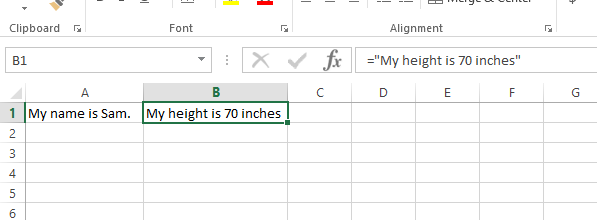But for statement a we cannot write like: =” My height is 70 ” ”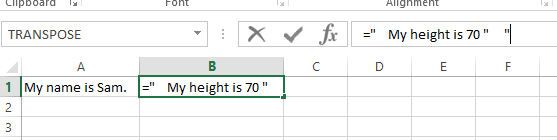It will return formula errors: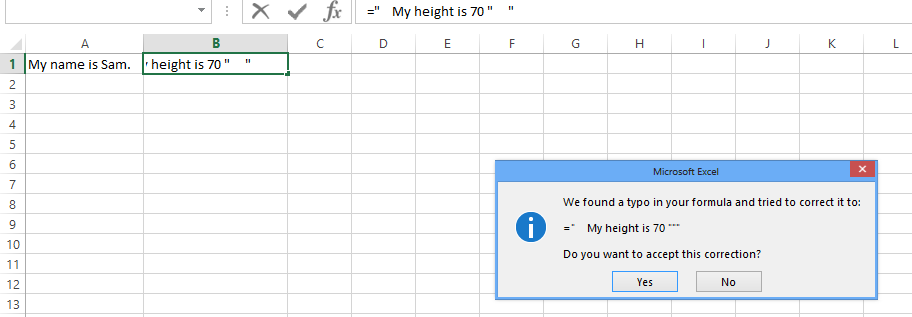So whenever we have quotes in a formula we have to understand that we have to escape the double quotes with double apostrophes to let Excel read it properly.

So the correct value: = ” My height is 70 “” ”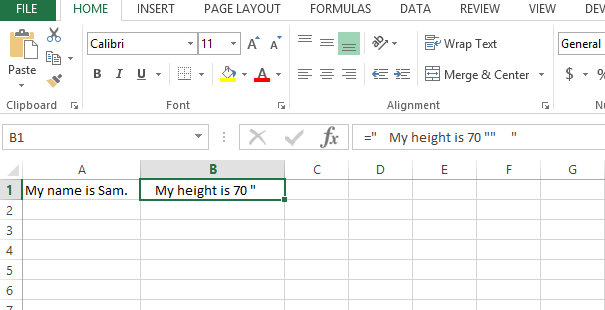### Using char 39

Another way to do this is using CHAR(39) for single quotes. The CHAR function allows you to enter a specific character code and display the corresponding character in a cell.

Like we used quotes before apostrophes in previous example, here we are using char(39) instead of writing the quotes:

=” My height is 70 ” & CHAR(39)

And the result will be same: My height is 70 ‘The code for a double quote is 34, so you can use the following formula to display a double quote:

=CHAR(34) & “Your text here” & CHAR(34)

### Using HTML code for a single quote

There is another method you can use to display a single quote in an Excel cell, which is to use the HTML code for a single quote, which is &#39;. To display a single quote, you can use the following formula:

=”Your text here &#39; single quote

For example, if you want to display the text “Don’t stop now” in a cell, you would enter the following formula:

=“Don&#39;t stop now”

The result in the cell will be:

Don’t stop now

### Using strings concatenation

Another method to display a single quote in an Excel cell is to use a formula that concatenates text strings. You can use the ampersand & operator to concatenate text strings in a formula. To display a single quote in a formula, you can use two double quotes together “” to represent a single quote.

For example, if you want to display the text “Don’t stop now” in a cell, you would enter the following formula:

=“Don” & “”“” & “t stop now”

The result in the cell will be:

Don‘t stop now

You can also use the concatenation formula to display other special characters, such as double quotes or new lines, in an Excel cell.

### Using ASCII code

Another method to display a single quote in an Excel cell is to use the ASCII code for a single quote, which is 39. You can use the CODE function to return the ASCII code for a character, and the CHAR function to return the character for a given ASCII code. To display a single quote, you can use the following formula:

=CHAR(CODE(“‘”)) & “Your text here” & CHAR(CODE(“‘”))

For example, if you want to display the text “Don’t stop now” in a cell, you would enter the following formula:

=CHAR(CODE(“‘”)) & “Don’t stop now” & CHAR(CODE(“‘”))

The result in the cell will be:

Don‘t stop now

### Using formula bar

Another method to display a single quote in an Excel cell is to use the Excel formula bar to enter the text. To do this, you can simply enter the text in the formula bar, rather than in the cell itself. To enter a single quote, you can press the single quote key on your keyboard.

For example, if you want to display the text “Don’t stop now” in a cell, you would do the following:

1. Select the cell in which you want to display the text.
2. Click on the formula bar above the worksheet to activate it.
3. Type the text Don’t stop now in the formula bar.
4. Press the Enter key to confirm the entry.

### Using TEXT function

Another method to display a single quote in an Excel cell is to use the formula text function. The text function allows you to format a number as text. To display a single quote in a formula, you can use the text function to format a blank text string as text.

For example, if you want to display the text “Don’t stop now” in a cell, you would enter the following formula:

=TEXT(“”,“Don'””t stop now”)

You can also use the text function to format numbers as text, and to apply custom formatting to numbers. The text function is useful for preventing unwanted changes to your data, such as the removal of leading zeros or the conversion of numbers to dates.

### Using REPT function

Another method to display a single quote in an Excel cell is to use the REPT function. The REPT function allows you to repeat a character or a string a specified number of times. To display a single quote, you can use the REPT function to repeat a single quote character.

For example, if you want to display the text “Don’t stop now” in a cell, you would enter the following formula:

=“Don” & REPT(“‘”,1) & “t stop now”

In conclusion, there are several methods for displaying a single quote in an Excel cell, including:

1. Using the CHAR function with the code for a single quote (39).
2. Using the formula bar to enter the text.
3. Using the TEXT function to format a number or text as text.
4. Using the REPT function to repeat a single quote character.
5. Using the HTML code for a single quote (`&#39;`).
6. Using the concatenation operator `&` to combine two or more strings.

Each of these methods has its own advantages and limitations, and the best method will depend on your specific needs and requirements. Regardless of the method you choose, the key is to understand how to properly enter the formula or text in the cell to ensure that the single quote is displayed correctly.